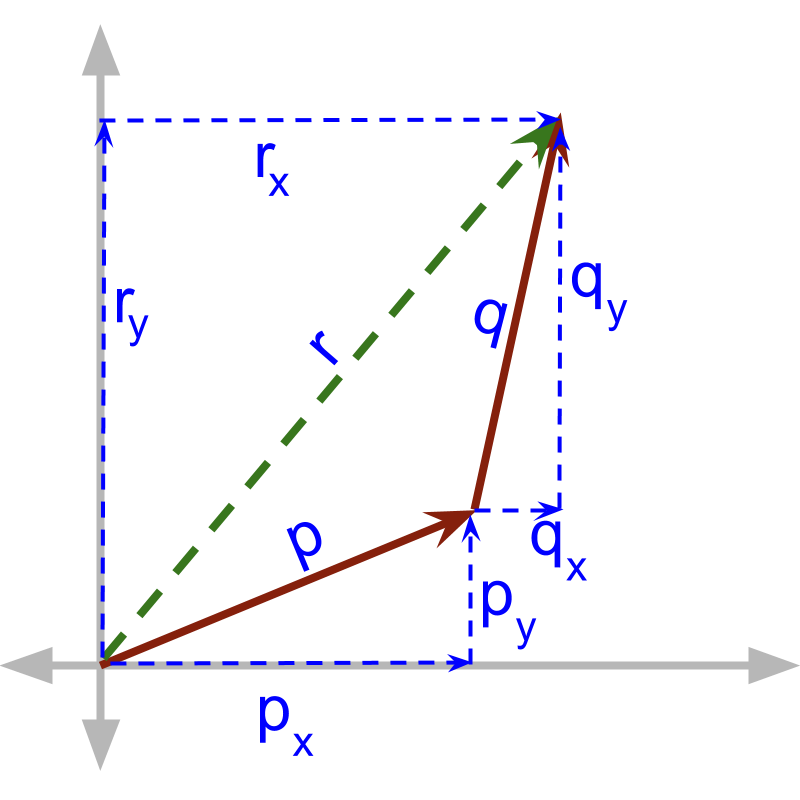maths > vector-algebra

what you'll learn...

Overview→  ${r}_{x}={p}_{x}+{q}_{x}$${r}_{x} = {p}_{x} + {q}_{x}$
→  ${r}_{y}={p}_{y}+{q}_{y}$${r}_{y} = {p}_{y} + {q}_{y}$
→  ${r}_{z}={p}_{z}+{q}_{z}$${r}_{z} = {p}_{z} + {q}_{z}$

component form

The component form of a vector is representation of vector with the 'components along $x,y,$$x , y ,$ and $z$$z$ axes'.

Two vectors $ai$$a i$ and $pi$$p i$ are added. The result of addition of the two vectors is $\left(a+p\right)i$$\left(a + p\right) i$
The magnitudes add up as two vectors are in the same direction $i$$i$ , that is, along x-axis.

The result of addition of two vectors $ai+bj$$a i + b j$ and $pi$$p i$ is '$\left(a+p\right)i+bj$$\left(a + p\right) i + b j$'. The magnitudes add up along the directions in parallel and the directions in perpendicular are kept separately. In this problem, in the direction of x-axis, $ai$$a i$ and $pi$$p i$ are given. They are added in magnitude.

That is expanded further. The result of addition of two vectors $ai+bj+cj$$a i + b j + c j$ and $pi+qj+rk$$p i + q j + r k$ is '$\left(a+p\right)i+\left(b+q\right)j+\left(c+r\right)k$$\left(a + p\right) i + \left(b + q\right) j + \left(c + r\right) k$'. The magnitudes add up along the directions in parallel and the directions in perpendicular are kept separately. In these two vectors, the components along the three axes are given in component form.

summary

Addition of two vectors: When two vectors $\stackrel{\to }{p}={p}_{x}i+{p}_{y}j+{p}_{z}k$$\vec{p} = {p}_{x} i + {p}_{y} j + {p}_{z} k$ and $\stackrel{\to }{q}={q}_{x}i+{q}_{y}j+{q}_{z}k$$\vec{q} = {q}_{x} i + {q}_{y} j + {q}_{z} k$ are added the result is $\stackrel{\to }{p}+\stackrel{\to }{q}=\left({p}_{x}+{q}_{x}\right)i$$\vec{p} + \vec{q} = \left({p}_{x} + {q}_{x}\right) i$$+\left({p}_{y}+{q}_{y}\right)j$$+ \left({p}_{y} + {q}_{y}\right) j$$+\left({p}_{z}+{q}_{z}\right)k$$+ \left({p}_{z} + {q}_{z}\right) k$

When vectors in the component form are added, the corresponding components are added.

Outline